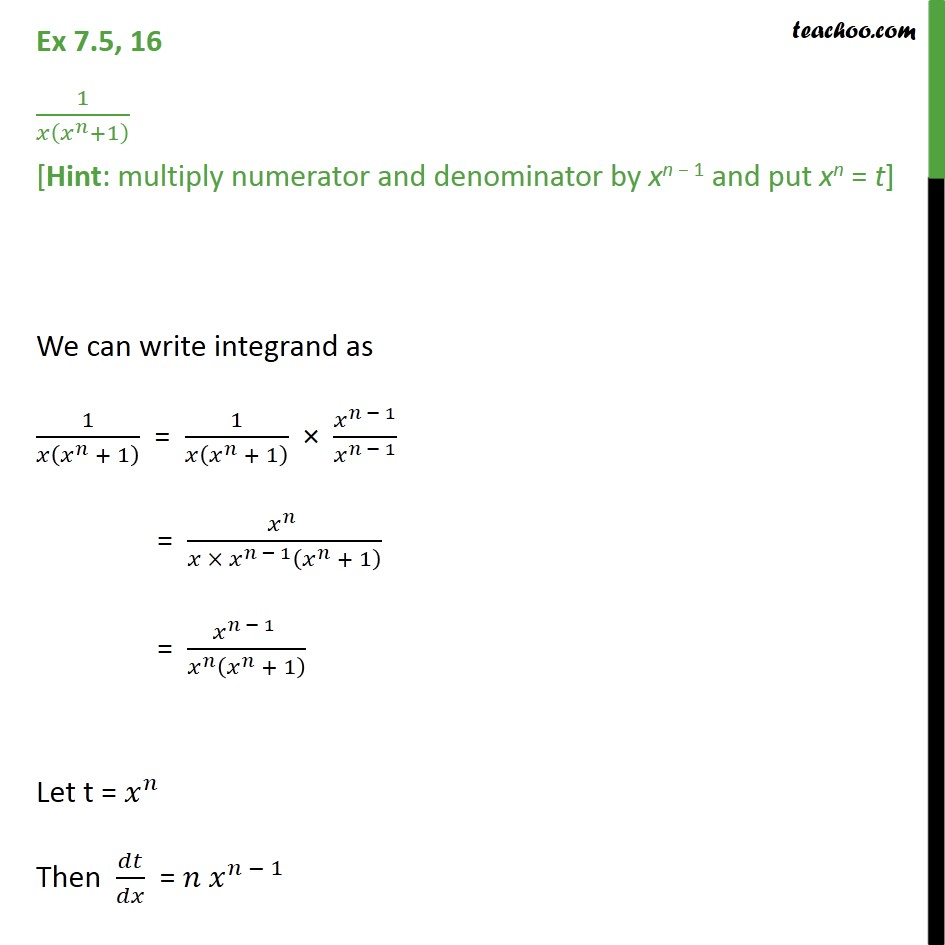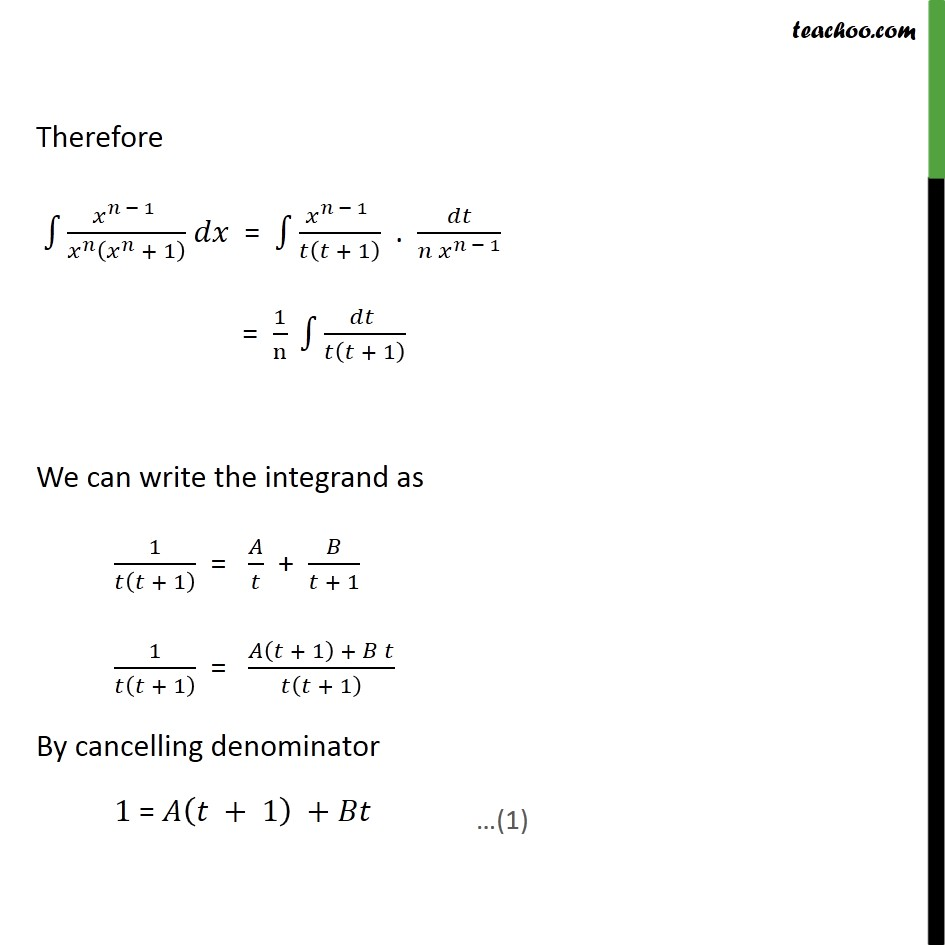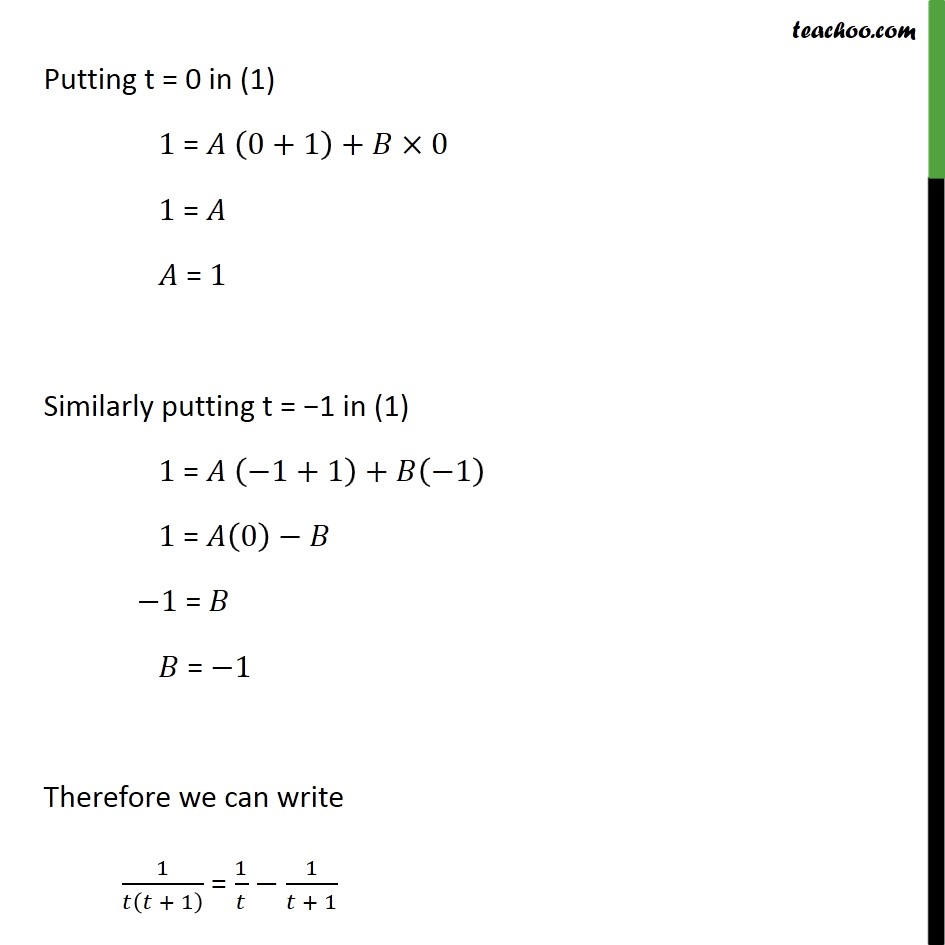Ex 7.5

Chapter 7 Class 12 Integrals
Serial order wiseLearn in your speed, with individual attention - Teachoo Maths 1-on-1 Class

### Transcript

Ex 7.5, 16 1 +1 [Hint: multiply numerator and denominator by xn 1 and put xn = t] We can write integrand as 1 + 1 = 1 + 1 1 1 = 1 + 1 = 1 + 1 Let t = Then = 1 Therefore 1 + 1 = 1 + 1 . 1 = 1 n + 1 We can write the integrand as 1 + 1 = + + 1 1 + 1 = + 1 + + 1 By cancelling denominator 1 = + 1 + Putting t = 0 in (1) 1 = 0+1 + 0 1 = = 1 Similarly putting t = 1 in (1) 1 = 1+1 + 1 1 = 0 1 = = 1 Therefore we can write 1 + 1 = 1 1 + 1 Thus, + 1 = 1 1 + 1 = log log +1 + C = log + 1 + Putting back t = 1 + 1 = + +